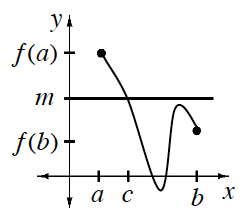### Home > APCALC > Chapter 3 > Lesson 3.1.2 > Problem3-27

3-27.

If $f(15) = -3$ and $f(20) = 4$, must $f$ have a root between $x = 15$ and $x = 20$? Explain why or why not. Be sure to include sketches that support your reasoning.Intermediate Value Theorem

Let $f$ be a function continuous on the closed interval $[a,b]$. Then for every value $m$ between $f (a)$ and $f (b)$ there exists a value $c$, $a < c < b$, such that $f (c) = m$.

Notice the condition of the Intermediate Value Theorem.